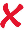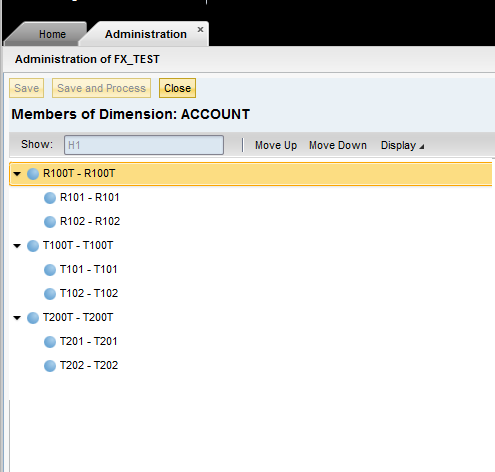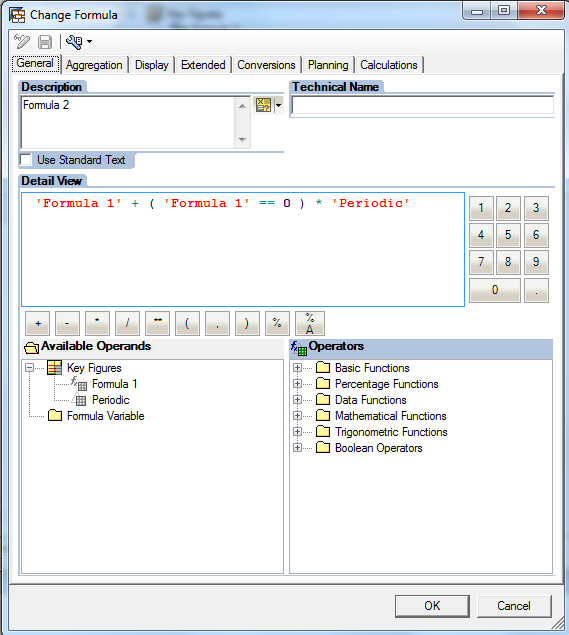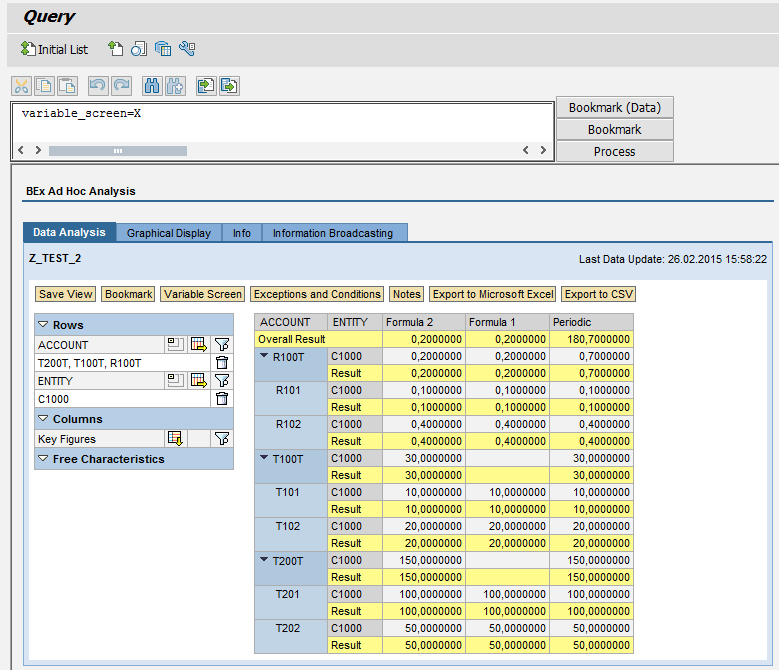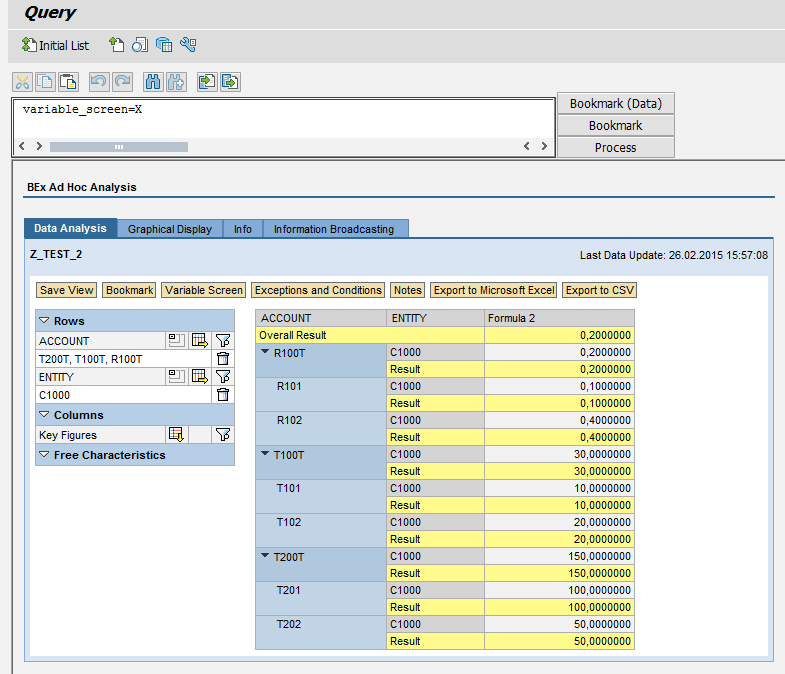# How to perform exception aggregation on BPC member

Member formula is widely used in BPC environment, but many customers facing the same challenge when running queries with aggregation on member formulas, the calculation result is incorrect. For example in following table, the correct result should be 0.2, but query result usually displayed as 0.5:

 ACCOUNT ENTITY PERIODIC explanation T100T C1000 30 T100T=SUM(T101, T102) T101 C1000 10 T102 C1000 20 T200T C1000 150 T100T=SUM(T101, T102) T201 C1000 100 T202 C1000 50 R100T C1000 R100T=T100T/T200T Not: SUM(R101, R102) R101 C1000 0.1 R101=T101/T201 R102 C1000 0.4 R102=T102/T202

This is a BPC production limitation at the moment, which is unable to handle exception aggregation on member formula for transient queries, and this can’t be handled in BW side alone either, as BW BEX query is unable to recognize the difference between member formula rows and normal rows.

The solution below is to combine both BPC member formula and BW exception aggregation methodology to handle this challenge for reporting.

1. Scenario

Here is account type, account hierarchy setup from BPC side:

R100T and its children nodes R101 and R102 are ratio nodes, which are calculated from T101, T201 etc.

1. Member formula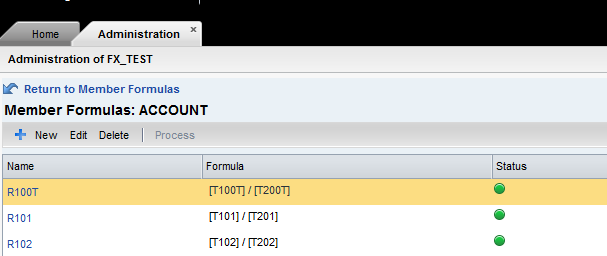R101 and R102 are member formula to calculate ratio. Besides the member formula on children nodes, also design accordingly member formula on parent node level (R100T = T100T/T200T).

1. Add “PERIODIC” as restricted key figure, and create “Formula 1” which is actually “PERIODIC”,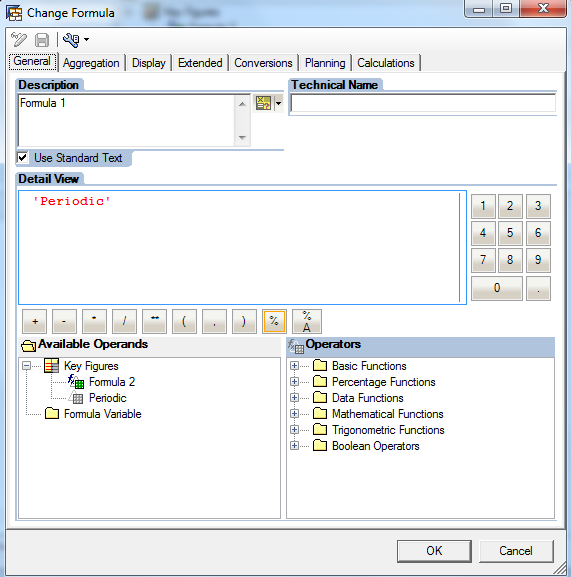1. Set the Exception Aggregation on “Formula 1”as “No aggregation along hierarchy”.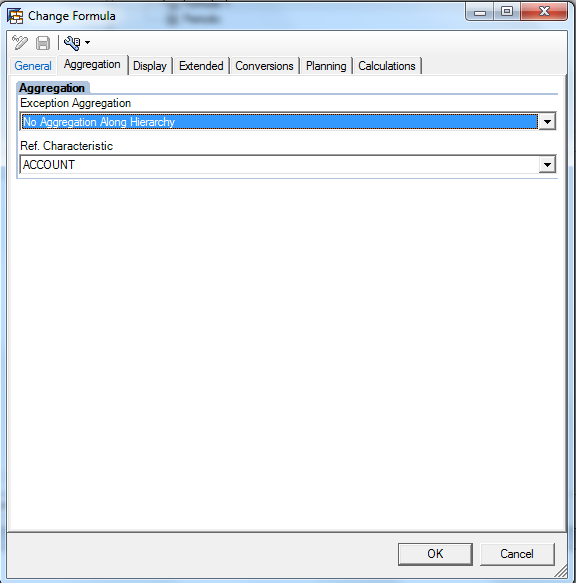1. Create “Formula 2” with following logic:

This formula is used to check the value for “Formula 1”, if “Formula 1” has a value (not empty),  then (“Formula 1” == 0) will return false value, which is 0, it’s going to use the value from “Formula 1”.  If “Formula 1” is empty ( because no aggregation on hierarchy for the exception aggregation),  then (“Formula 1” == 0) will return true value, which is 1, the value from “Periodic” will used for the final result.

1. Use standard aggregation on “Formula 2”(no exception aggregation)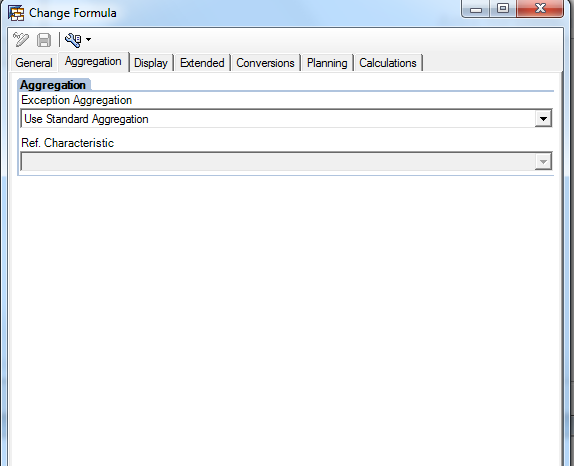1. Run the BEX query, you will find the R100T aggregation on:

“Periodic” is 0.7 (incorrect), which is a result of 0.2(number calculated from member formula in BPC) + 0.5 (aggregation result from BW, 0.1 +0.4) “Formula 1” on R100T is correct, 0.2(number calculated from member formula in BPC), but it’s empty for T100T, T200T, because we set exception aggregation on BW ( No aggregation on hierarchy).“Formula 2” is correct for all aggregation nodes, because we used formula in BEX query.(if “Formula 1” is empty, take the value from original aggregation value from “Periodic”.

1. Now hide the key figures “Periodic” and “Formula 1”,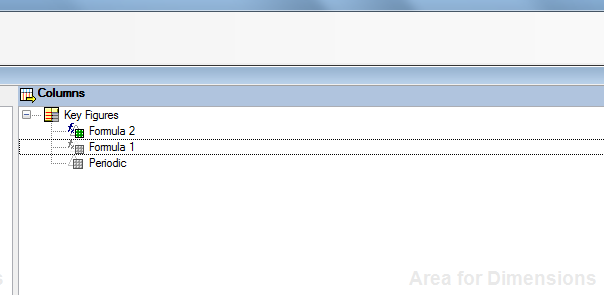The query result will look like below, correct aggregation numbers will be displayed for all nodes:

Above is just an example for hierarchy with periodic, similar method could be used for YTD/QTD calculations like:

 2014.01 2014.02 2014.03 2014.Q1 A 10 20 30 60 B 5 8 6 19 C=A/B 2 2.5 5 ?

Feedback:

If you have any comments, questions or feedback, please kindly send an e-mail to:

George Yang (ge.yang@sap.com)

Freeter Xie (freeter.xie@sap.com)

Alex Shen (yang.shen@sap.com)

/